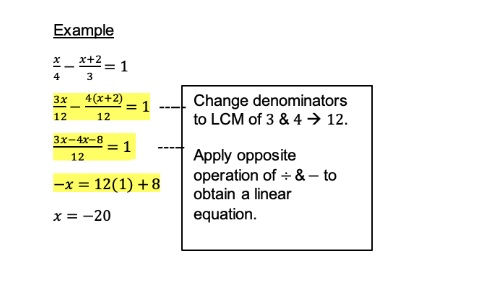top of page
Search

# 5 Common Mistakes in Secondary Math Linear Equations Problems and How to Avoid ThemLinear equations are a fundamental concept in secondary math, yet they can be a source of frustration for many students. From misinterpreting the problem to miscalculating the solution, mistakes can easily derail your progress.

Fortunately, by understanding the most common mistakes and how to avoid them, your child can succeed in this crucial area of study. In this article, we'll highlight five common mistakes in solving systems of linear equations for secondary school and provide useful strategies to help you overcome them.

## What are Linear Equations?

Linear equations are a fundamental component of secondary-level math, allowing us to establish the relationship between two variables. Essentially, linear equations represent a straight line on a graph, helping us understand the correlation between two variables.

Nonetheless, many students struggle with solving linear equations and often make mistakes in Secondary Math exams, which can be disappointing.

## 5 Common Mistakes in Secondary Math Linear Equations Problems and How to Correct Them### 1. Not Performing the Same Operation on Both Sides of the Equation

When solving linear equations, students often overlook the importance of applying the same operation to both sides of the equation. This oversight can lead to an unbalanced equation and an incorrect solution. It is crucial to remember that any operation performed on one side of the equation must be done on the other side to maintain balance and ensure the accuracy of the solution.

To avoid this mistake, always perform the same operation on both sides of the equation. Whether adding or subtracting a value, multiplying, or dividing both sides by a value, maintaining symmetry is key to obtaining the correct solution.### 2. Misapplying the Distributive Property

A common mistake students make while solving linear equations is misusing the distributive property. This mathematical rule involves multiplying a value outside the parentheses with each term inside the parentheses.

However, students may incorrectly apply this rule, leading to an unbalanced equation and an inaccurate solution. Therefore, it is vital to double-check the distributive property and ensure that it is applied correctly.

To avoid this mistake, always remember to correctly distribute the value outside the brackets by multiplying it with each term inside the brackets. Take care to perform the multiplication accurately for each term to maintain the balance of the equation and obtain the correct solution.### 3. Making Errors When Combining Like Terms With Negative Signs

When solving linear equations, students may encounter errors when combining like terms with negative signs. This mistake can occur when students fail to identify the negative signs of each term and incorrectly combine them. This error can lead to an unbalanced equation and an inaccurate solution. Therefore, it is crucial to be mindful of the negative signs when combining like terms and double-check the equation's balance.

To avoid this mistake, it is important not to skip steps while working through the problem. Take the time to carefully identify the terms with the same variable and exponent and ensure that the signs are correct when combining them.

After obtaining a solution, it is recommended to substitute the final value back into the initial equation to double-check if the answer is correct. This step helps verify the accuracy of the solution and confirms that it satisfies the original equation.### 4. Error in Applying the Opposite Operation of Division and Multiplication

Misapplying the opposite operation of division and multiplication is a common mistake when solving linear equations. This error occurs when students forget to use brackets while applying the opposite operation, resulting in incorrect intermediary steps and, ultimately, an incorrect answer. Therefore, it is essential to be mindful of the order of operations and apply the inverse operation correctly.

To avoid this mistake, it is important to add parentheses to the right-hand side (RHS) of the equation before applying the opposite operation of division and multiplication. This ensures that the operation is performed on the entire expression within the parentheses, maintaining the integrity of the equation. By using parentheses, you can accurately apply the opposite operation and prevent mistakes in your solution.### 5. Not Converting Fractional Equations Into Linear Equations First Before Solving

Converting fractional equations into linear equations is a crucial step in solving linear equations problems that involve fractions. This conversion enables easier manipulation and simplification of the equation, leading to accurate solutions.

Neglecting this step can result in an unbalanced equation and incorrect solutions. Therefore, it is essential to convert fractional equations into linear equations before proceeding with the solution.

To convert a fractional equation into a linear equation, start by finding the least common multiple (LCM) of the denominators. Then, multiply both sides of the equation by the LCM to eliminate the fractions. This step ensures that the equation becomes linear, allowing for straightforward solving.Knowing the common mistakes in solving linear equations and how to avoid them is crucial for success in secondary math. By applying the strategies discussed in this article, such as performing the same operation on both sides of the equation and converting fractional equations into linear equations first, students can improve their accuracy and confidence in solving linear equations during exams.At AGrader Learning Centre, we are dedicated to helping your child build a strong foundation and achieve top grades. Our experienced team has developed a curriculum that aligns with the latest Ministry of Education standards, ensuring your child excels in math and achieves better scores.

We provide carefully designed materials and worksheets in our Secondary Math Tuition lessons, giving students a clear understanding of what to expect in exams and boosting their confidence and preparedness.